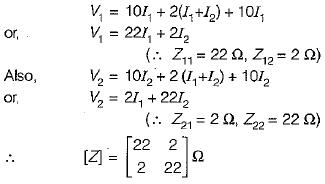Courses

# Two Port Network

## 10 Questions MCQ Test Topicwise Question Bank for Electronics Engineering | Two Port Network

Description
This mock test of Two Port Network for Electrical Engineering (EE) helps you for every Electrical Engineering (EE) entrance exam. This contains 10 Multiple Choice Questions for Electrical Engineering (EE) Two Port Network (mcq) to study with solutions a complete question bank. The solved questions answers in this Two Port Network quiz give you a good mix of easy questions and tough questions. Electrical Engineering (EE) students definitely take this Two Port Network exercise for a better result in the exam. You can find other Two Port Network extra questions, long questions & short questions for Electrical Engineering (EE) on EduRev as well by searching above.
QUESTION: 1

### The two port matrix of an 1 : n ideal transfer is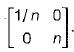It describes the transfer in terms of its

Solution:

An ideal transfer having turns ratio of 1 : n is shown below.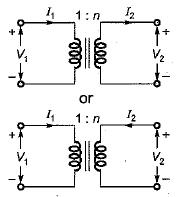Here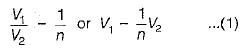Also,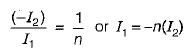or,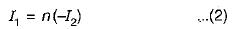Comparing equations (1) and (2) with standard transmission-parameter equations,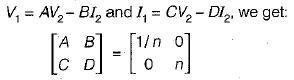QUESTION: 2

Solution:
QUESTION: 3

### A two port network is not reciprocal if

Solution:
QUESTION: 4

Assertion (A): Unilateral elements offer varying impedances with variations in flow of current while bilateral elements offer same impedance irrespective of flow of current.

Reason (R): If the magnitude of the current passing through an element is affected due to change in the polarity of the applied voltage, the element is called unilateral element while if the current magnitude remains the same even if the applied emf’s polarity is changed, it is called a bilateral element

Solution:
QUESTION: 5

The  parameters Z11 a nd Z22 of the given T-network shown below are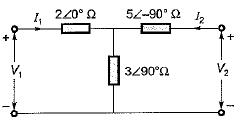Solution: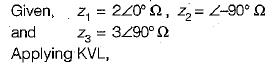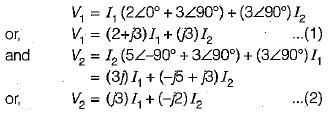Comparing equations (1) and (2) with the standard z-parameter equations;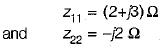QUESTION: 6

Match List-1 with List-11 and select the correct answer using the codes given below the lists: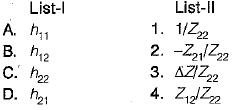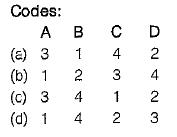Solution:

Z-parameter equations are: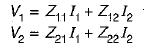and h parameter equations are: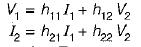Converting the Z-parameter equations into h-parameter equations, we have: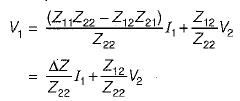and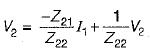QUESTION: 7

in a two port network, Y11 is given by

Solution: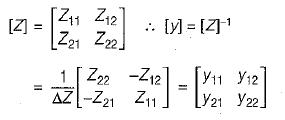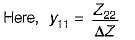QUESTION: 8

The value of Z21(s) in the circuit shown below is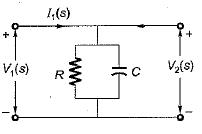Solution:

input admittance,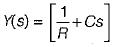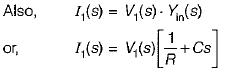As V1(s) = V2{s) therefore,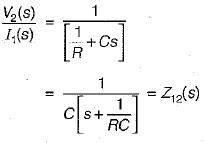QUESTION: 9

A two port network has zero initial conditions. At t = 0; an impulse voltage δ(t) is applied at the input producing a voltage e-2t + e-3t)  u(t) volts at the output. If the output voltage required is te-2t u(t) then, the input voltage will be

Solution:

Given:
Input voltge,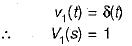and output,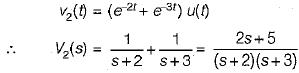Now, impulse response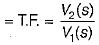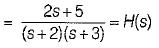Given, output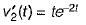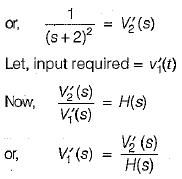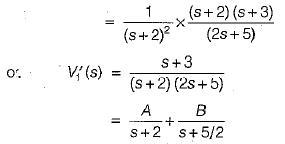Using partial fraction expansion, A = 1 and B = -0.5.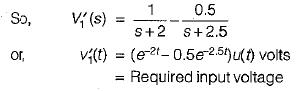QUESTION: 10

The Z-parameter of the two port network shown below is given by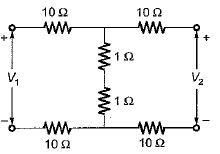Solution: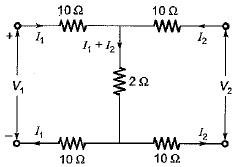Applying KVL in the two (oops,